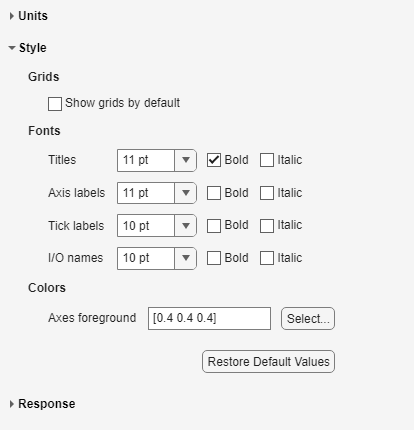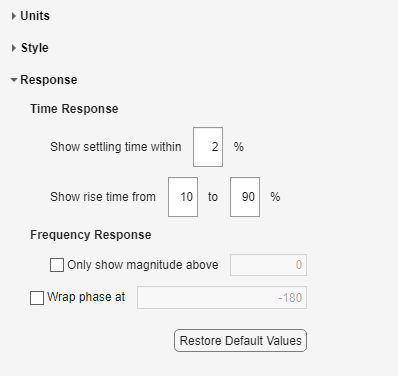Documentation

## Toolbox Preferences Editor

### Overview of the Toolbox Preferences Editor

The Toolbox Preferences editor allows you to set plot preferences that will persist from session to session.

### Opening the Toolbox Preferences Editor

To open the Toolbox Preferences editor, select Toolbox Preferences from the File menu of the Linear System Analyzer or the Control System Designer. Alternatively, you can type

`identpref`

at the MATLAB® prompt.

Control System Toolbox Preferences Editor### Note

Control System Designer requires the Control System Toolbox™ software.

### Units PaneUse the Units pane to set preferences for the following:

• Frequency

The default `auto` option uses `rad/TimeUnit` as the frequency units relative to the system time units, where `TimeUnit` is the system time units specified in the `TimeUnit` property of the system on frequency-domain plots. For multiple systems with different time units, the units of the first system is used.

For the frequency axis, you can select logarithmic or linear scales.

• Magnitude — Decibels (dB) or absolute value (abs)

• Phase — Degrees or radians

• Time

The default `auto` option uses the time units specified in the `TimeUnit` property of the system on the time- and frequency-domain plots. For multiple systems with different time units, the units of the first system is used.

### Style Pane

Use the Style pane to toggle grid visibility and set font preferences and axes foreground colors for all plots you create. This figure shows the Style pane.You have the following choices:

• Grid — Activate grids by default in new plots.

• Fonts — Set the font size, weight (bold), and angle (italic). Select font sizes from the menus or type any font-size values in the fields.

• Colors — Specify the color vector to use for the axes foreground, which includes the X-Y axes, grid lines, and tick labels. Use a three-element vector to represent red, green, and blue (RGB) values. Vector element values can range from 0 to 1.

If you do not want to specify RGB values numerically, click the Select button to open the Select Colors dialog box.

### Options Pane

The Options pane has selections for time responses and frequency responses. This figure shows the Options pane with default settings.For time response plots, the following options are available:

• Show settling time within xx% — Set the threshold of the settling time calculation to any percentage from 0 to 100%. The default is 2%.

• Specify rise time from xx% to yy%— The standard definition of rise time is the time it takes the signal to go from 10% to 90% of the final value. Specify any percentages you like (from 0% to 100%), provided that the first value is smaller than the second.

For frequency response plots, the following options are available:

• Only show magnitude above — Specify a lower limit for magnitude values in response plots so that you can focus on a region of interest.

• Wrap phase — Wrap the phase into the interval [–180º,180º). To wrap accumulated phase at a different value, enter the value in the Branch field. For example, entering 0 causes the plot to wrap the phase into the interval [0º,360º).

### Control System Designer Pane

The Control System Designer pane has settings for Control System Designer. This figure shows the Control System Designer pane with default settings.You can make the following selections:

• Compensator Format — Select the time constant, natural frequency, or zero/pole/gain format. The time constant format is a factorization of the compensator transfer function of the form

`$K×\frac{\left(1+{T}_{{z}_{1}}s\right)\left(1+{T}_{{z}_{2}}s\right)}{\left(1+{T}_{{p}_{1}}s\right)\left(1+{T}_{{p}_{2}}s\right)}\cdots$`

where K is compensator DC gain, Tz1, Tz2, ..., are the zero time constants, and Tp1, Tp2, ..., are the pole time constants.

The natural frequency format is

`$K×\frac{\left(1+s/{\omega }_{{z}_{1}}\right)\left(1+s/{\omega }_{{z}_{2}}\right)}{\left(1+s/{\omega }_{{p}_{1}}\right)\left(1+s/{\omega }_{{p}_{2}}\right)}\cdots$`

where K is compensator DC gain, ωz1, and ωz2, ... and ωp1, ωp2, ..., are the natural frequencies of the zeros and poles, respectively.

The zero/pole/gain format is

`$K×\frac{\left(s+{z}_{1}\right)\left(s+{z}_{2}\right)}{\left(s+{p}_{1}\right)\left(s+{p}_{2}\right)}$`

where K is the overall compensator gain, and z1, z2, ... and p1, p2, ..., are the zero and pole locations, respectively.

• Bode Options — By default, the Control System Designer shows the plant and sensor poles and zeros as blue x's and o's, respectively. Clear this box to eliminate the plant's poles and zeros from the Bode plot. Note that the compensator poles and zeros (in red) will still appear.

##### Support